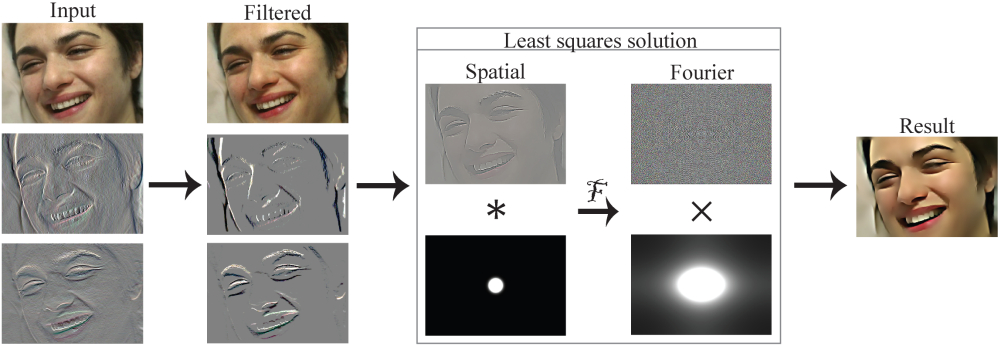Fourier Analysis of the 2D Screened Poisson Equation for Gradient Domain Problems Abstract We analyze the problem of reconstructing a 2D function that approximates a set of desired gradients and a data term. The combined data and gradient terms enable operations like modifying the gradients of an image while staying close to the original image. Starting with a variational formulation, we arrive at the 'screened Poisson equation' known in physics. Analysis of this equation in the Fourier domain leads to a direct, exact, and efficient solution to the problem. Further analysis reveals the structure of the spatial filters that solve the 2D screened Poisson equation and shows gradient scaling to be a well-defined sharpen filter that generalizes Laplacian sharpening, which itself can be mapped to gradient domain filtering. Results using a DCT-based screened Poisson solver are demonstrated on several applications including image blending for panoramas, image sharpening, and de-blocking of compressed images.Citation   Bhat P., Curless B., Cohen M., and Zitnick L. Fourier Analysis of the 2D Screened Poisson Equation for Gradient Domain Problems. European Conference on Computer Vision (ECCV) 2008. Bibtex     Downloads   ECCV Paper - (PDF) ECCV Poster - (PDF) Code for the Fourier solver - (CPP)     Related projects   GradientShop - (website)